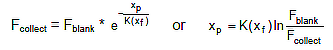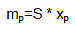# Beta Attenuation Technique

The mass determination principle is based on the physical law whereby beta rays are attenuated as they pass through a thin layer of material.

If one defines xf as the value of the filter's mass density and xp as the value of the mass density of the collected particles, the following equation applies:where K(xf) = mass absorption coefficient and Fblank and Fcollect represent the beta ray flow before and after the sampling cycle.

Using the value of the sampling surface area S (=11.95 cm², O = 3.9 cm), the mass of the suspended particles mp deposited on the filter can be computed as follows:The function K(xf) was determined by the instrument manufacturer and is programmed into the system. After each power-up of the instrument, the stability of the initial calibration is verified by means of two reference orifices placed in the passage of the beta rays between the source and the Geiger counter. This function can also be triggered manually and is available as a regular Auto Test feature. The result of the last "beta test" can be viewed.

## Product

SM200 Particulate Monitor >>